# Sets

A mathematical set is defined as an unordered collection of elements. On the GMAT, sets typically are comprised of numbers, either enumerated within the curly brackets { } or described in words. For example, the set S = {1, 2, 3} contains 1, 2, and 3 as members.

For a set A having an element x, the following are all used synonymously:

x is a member of A

x is contained in A

x is included in A

x is an element of the set A

A contains x

A includes x

Sets are unordered. The expressions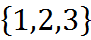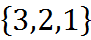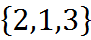all specify the same set.

A set S is a subset of set A if every member of S is a member of A. We use the horseshoe notation to indicate subsets. The expression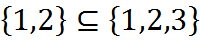says that {1,2} is a subset of {1,2,3}.

## Union and Intersection

The union of two sets A and B, written with a shape that looks like a capital “U,” is the set that contains all the members of A and all the members of B (and nothing else). That is,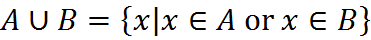As an example,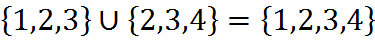The intersection of two sets A and B, written with a shape that looks like a lowercase “n” (for iNtersection), is the set that contains everything that is a member of both A and B (and nothing else). That is,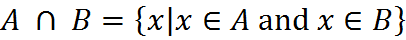This set notation is technically included in what you should know about sets, but it appears rarely on GMAT questions.

For example,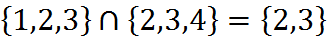Two sets are disjoint or mutually exclusive if their intersection is empty. Some GMAT questions will hinge on whether two sets overlap or not – that is, whether they have an intersection or are disjoint.

## Number of Elements

The number of elements in a set is indicated by vertical bars (not curly brackets). So if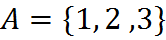then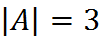because the set A includes three elements. Although this notation uses the same marks as absolute value, the two uses of the symbols are not related.

The official language about the GMAT mentions what it calls the “general addition rule for two sets,” which it gives in set terminology as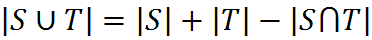This fact is central to many GMAT questions. Here we’ll cover a version of the equation that’s both easier to remember and more powerful – we will call it the Overlapping Set Equation – but first, we will review Venn diagrams.

Welcome! You are encouraged to register with the site and login (for free). When you register, you support the site and your question history is saved.